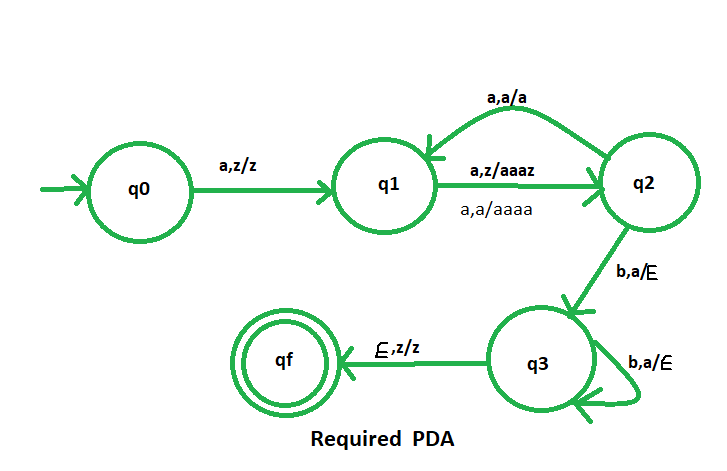Open in App
Not now

# NPDA for accepting the language L = {a2mb3m | m ≥ 1}

• Difficulty Level : Easy
• Last Updated : 28 Aug, 2019

Prerequisite – Pushdown automata, Pushdown automata acceptance by final state
Problem – Design a non deterministic PDA for accepting the language L = {a2mb3m | m ≥ 1}, i.e.,

L = {aabbb, aaaabbbbbb, aaaaaabbbbbbbbb, aaaaaaaabbbbbbbbbbbb, ......}

In each of the string, for every 2 ‘a’s there is 3 ‘b’.

Explanation –
Here, we need to maintain the order of a’s and b’s.That is, all the a’s are coming first and then all the b’s are coming. Thus, we need a stack along with the state diagram. The count of a’s and b’s is maintained by the stack. Here, we have 3 ‘b’s for every 2 ‘a’s. We will take 2 stack alphabets:= {a, z}
Where,= set of all the stack alphabet
z = stack start symbol 

Approach used in the construction of PDA –
As we want to design a NPDA, thus every time ‘a’ comes before ‘b’. We will push three ‘a’s into the stack for two consecutive two ‘a’s and again for the next two ‘a’s, we will push three ‘a’ into the stack. That is, for the first ‘a’ we will do nothing only state will change and for the next ‘a’ we will do the pushing operation and similarly we perform this alternatively, i.e.,
For two a’s we push three ‘a’
For four b’s we push six ‘a’
After that, when ‘b’ comes then pop one ‘a’ from the stack each time.

So, at the end if the stack becomes empty then we can say that the string is accepted by the PDA.

Stack transition functions –(q0, a, z)(q1, z)     [ Indicates no operation only state change ](q1, a, z)(q2, aaaz)  [ Indicates push operation for alternate 'a'](q2, a, aaaz)(q1, aaaz) [ Indicates no operation only state change ](q1, a, aaaz)(q2, aaaa) [ Indicates push operation for alternate 'a'](q2, b, a)(q3,)  [Indicates pop operation ](q3, b, a)(q3,)  [Indicates pop operation ](q3,, z)(qf, z )


Where, q0 = Initial state
qf = Final state= indicates pop operation

Correct transition diagram-My Personal Notes arrow_drop_up
Related Articles